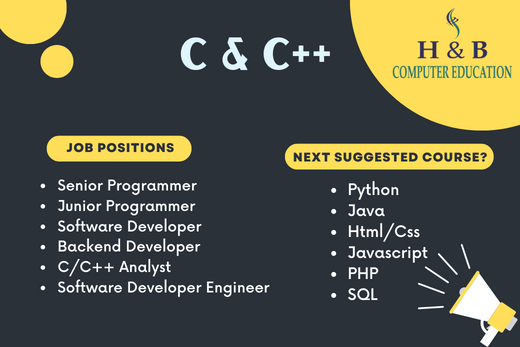# C , C++## Course Overview

C++ is an object-oriented programming (OOP) language that is viewed by many as the best language for creating large-scale applications. C++ is a superset of the C language.

A related programming language, Java, is based on C++ but optimized for the distribution of program objects in a network such as the Internet. Java is somewhat simpler and easier to learn than C++ and has characteristics that give it other advantages over C++. However, both languages require a considerable amount of study.

## Course lessons

INTRO C
BASIC C
C INSTALL
TOKENS
VARIABLE

1. OPERATOR
• UNARY
• INCREMENT
• DECREMENT
• BINARY
• ARITHMETIC
• ASSIGNMENT
• RELATIONAL
• LOGICAL

2. CONDITIONAL STATEMENT
• IF
• IF ELSE
• IF ELSE IF ELSE
• NESTED IF ELSE
• SWITCH CASE

3. JUMP STATEMENT
• BREAK
• CONTINUE
• GOTO

4. LOOP
• WHILE
• DO WHILE
• FOR

5. ARRAY
• one dimantional
• two dimantional

6. STRING FUNCTION

7. MATH FUNCTION

8. STRUCTURE

9. POINTER

10.USER DEFINED FUNCTIONS
• NO ARGUMENT AND NO RETURN
• NO ARGUMENT AND WITH RETURN
• WITH ARGUMENT AND NO RETURN
• WITH ARGUMENT AND WITH RETURN

11. Recursion
INTRO C
BASIC C
C INSTALL
TOKENS
VARIABLE

1. OPERATOR
• UNARY
• INCREMENT
• DECREMENT
• BINARY
• ARITHMETIC
• ASSIGNMENT
• RELATIONAL
• LOGICAL

2. CONDITIONAL STATEMENT
• IF
• IF ELSE
• IF ELSE IF ELSE
• NESTED IF ELSE
• SWITCH CASE

3. JUMP STATEMENT
• BREAK
• CONTINUE
• GOTO

4. LOOP
• WHILE
• DO WHILE
• FOR

5. ARRAY
• one dimantional
• two dimantional

6. STRUCTURE

7. POINTER

8. USER DEFINED FUNCTIONS
• NO ARGUMENT AND NO RETURN
• NO ARGUMENT AND WITH RETURN
• WITH ARGUMENT AND NO RETURN
• WITH ARGUMENT AND WITH RETURN

9. Recursion

10. OOPS
• CLASS
• OBJECT
• CONSTRUCTOR
• FUNCTION OVERRIDING
•  INHERITANCE
• POLYMOREPYSM
• ENCAPSULATION
• DATA ABSTRACTION
• VIRTUAL FUNCTION
• FRIEND FUNCTION

#### H & B

Computer Education